## Solution - NAFEMS Benchmark Challenge 03

### January 18th 2015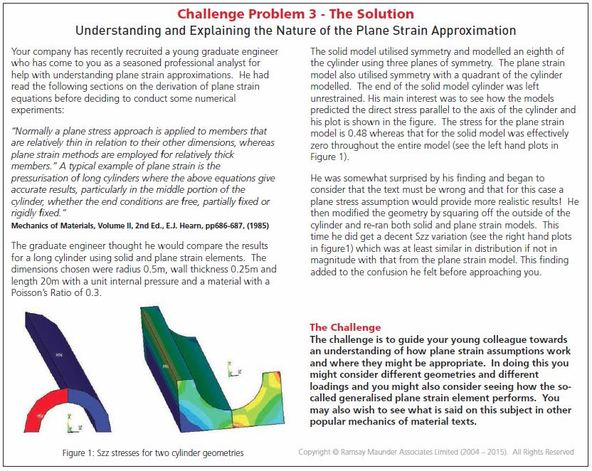## Raison d’être for the Challenge

There is much to be said about using plane stress or plane strain two-dimensional approximations for a three dimensional problem, not least the simplification incurred by this reduction in dimension. However in order to be able to use these approximations, the engineer needs to be confident about the limitations of the methods and when they might or might not be applicable. It seems from this challenge that not all is clear in this area and this response will use the finite element method to conduct a practical, rather than theoretical, exploration of this issue and hopefully identify what is happening and provide some useful rules that the practising engineer might consider.

## Cylinder Geometry

In Hearn’s text, as quoted in the challenge, he explicitly states that he is considering the case of pressurised long cylinders. There is some ambiguity here since the formal definition of a cylinder includes both circular and elliptical cross sections although it is understood that he probably meant circular geometries. In posing the challenge it was demonstrated that, for the circular cylinder with free ends (one of the conditions mentioned by Hearn), the longitudinal stress was essentially zero and thus, therefore, corresponding to a plane-stress form of solution. The challenge then looked at a different prismatic geometry; that of a square with a central circular hole. Whilst strictly not a cylinder, this problem showed a significant longitudinal stress when the end faces were free and therefore corresponded more with the idea of a plane strain scenario. Whilst provided the configuration is prismatic it may be considered as a planar problem, we will, to conform with Hearn’s definition, consider only cylindrical geometries and replace the problem of a square with a central hole with an elliptical cylinder.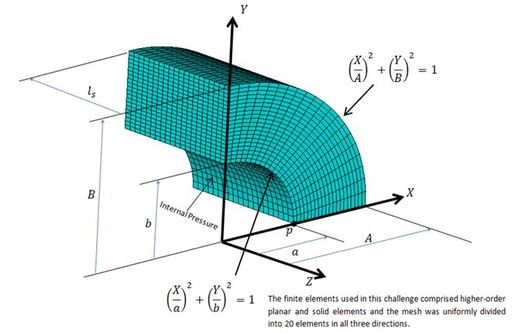Figure 2:  Geometry of bodies considered

The problem(s) considered are defined in figure 2 which shows a symmetrical eighth of a cylinder (symmetry about the three Cartesian planes, X=0, Y=0 and Z=0). The inner and outer curves are defined in the figure as ellipses and the coefficients used are shown in table 1.Note that when the coefficients for a curve are identical then the ellipse degenerates into a circle. The semi length,l_s, is half the total length of the body. The point p, which will be used to monitor stress values, lies at the position {A, 0, 0}.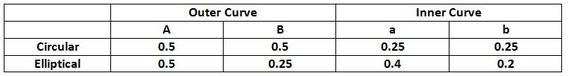Table 1:  Curve coefficients (m) for bodies considered

## Planar Solutions

Let us start off this study by looking at the stress fields for the circular and elliptical cylinders using the plane stress and plane strain approximations.

The stress contours shown in figure 3 are for the plane strain assumption where we are expecting non-zero axial stress as seen. Similar analyses were conducted using the plane stress assumption and whilst the axial stress was then zero, the stresses in the plane were identical to those generated with the plane strain assumption. This may or may not be surprising to the reader, but the theoretical solution to such problems when the applied body forces are zero (as in this case) involves in-plane stresses that are independent of the elastic constants (Young’s Modulus and Poisson’s Ratio). The axial stress however is strongly dependent on the value of Poisson’s Ratio such that if this property is zero then no axial strain will be developed and, therefore, for the case of plane strain, no axial stress.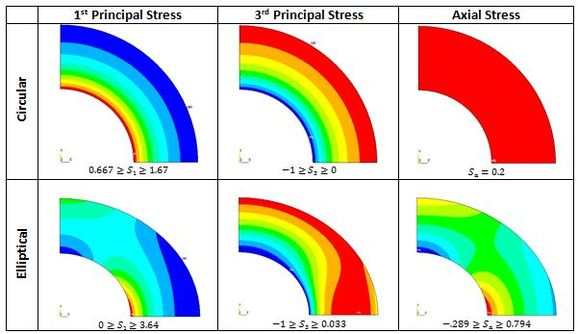Figure 3:  Stress contours for a Plane Strain assumption (Poisson’s Ratio = 0.3)

The minimum and maximum values of the stress normalised (divided) by the applied internal pressure are also shown in figure 3. For the circular geometry the 1st and 3rd principal stresses are seen to be identical, respectively, to the hoop and radial stresses as given by the Lamé equations for thick pressure vessels.The maximum radial stress is equal to the applied pressure at the inner curve and to zero at the outer curve.The maximum hoop stress is greater than the peak radial stress by a factor of about 1.67 and the axial stress, which is tensile, is about 20% of the applied pressure and is constant over the plane. For the elliptical geometry, where the 3rd principal stress is equal to the applied pressure on the inner curve, the amplification of the maximum principal stress is greater than that for the circular case at about 3.64. The axial stress for the elliptical case is no longer constant, showing a variation that now includes both tensile and compressive portions.However, if the stress were integrated over the area then it will be the case that the net axial force is tensile.

## Three Dimensional Solutions

The case of plane strain occurs when, for a three-dimensional body, the end faces are fixed. In addition, as will be shown later, this is independent of the length of the body. If the end faces are not fixed then the axial stresses on these faces are theoretically zero (although conventional finite elements will only show this when the mesh is sufficiently well refined). In the interior of the body, say at the centre plane, they may not be zero although they will, by definition, form a self balancing set, i.e. they will have no resultant force.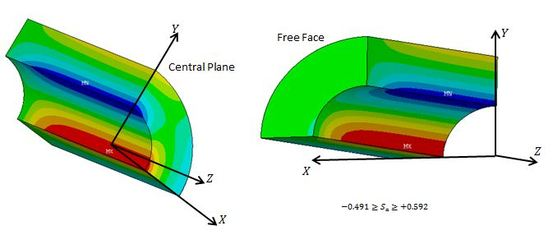In figure 4 the contours of the axial stress component are plotted for the elliptical cylinder with free ends. It is clearly seen how, whilst the axial stress at the central plane (Z=0) is non-zero, it decays towards the free end (Z=-l_s) so that the field is, approximately, zero. This is as expected and explained earlier. The axial stress contours on the central plane,whilst looking similar to those of the plane stress model, have different values, as indicated in the figure, and now integrate to a zero resultant force across the face.

The self-balancing axial stress field occurs as a direct result of the in-plane strains and Poisson’s ratio; if Poisson’s ratio were set to zero then the axial stresses would be zero. For the circular cylinder we have already seen (figure 1) that no significant axial stresses are developed. For this case the body has axial symmetry (about the Z axis) and the in-plane strains lead to a constant strain in the axial direction and zero axial stress. The corresponding strain for the elliptical geometry is not constant and leads to the development of internal self-balancing axial stress distributions even if the body is free in the axial direction. Thus one might consider the development of axial stress in the free case to be a result of internal restraint as opposed to the external restraint which occurs for the fixed case.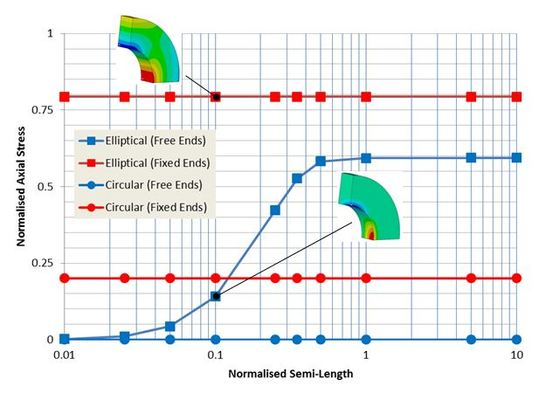Figure 5: Axial stress (maximum) as a function of semi-length

In figure 5 we have plotted the maximum axial stress (occurring at point p) as a function of the semi-length. Both quantities have been normalised, the stress by the applied pressure and the semi-length by the maximum semi-axis of the cross section (A).

The curves representing the circular geometry show an invariance of axial stress with body length for both free and fixed cases. Thus, as seen in this challenge, the plane stress model is appropriate for the free case irrespective of the length of the body. In contrast, for the elliptical geometry, whereas plane stress is appropriate for the free case only when the length of the body is small, as the length increases self-balancing axial stresses are generated (by internal restraint) with the maximum axial stress converging (with body length) to about 0.75 of the value for the fixed case. These are not the same results as would be obtained by the plane strain approximation but this form of approximation is clearly more appropriate than would be that of plane stress.

For the elliptical geometry considered here, the normalised axial length required for the self-balancing axial stress field to fully develop is about unity as indicated in figure 5. When the body is shorter than this there is insufficient length for the full development of the axial stress and thus the peak value decreases with length.Below a normalised length of about 0.05 the axial stresses are insignificant and a plane stress approximation would provide a reasonable representation of the state of stress.

## Closure

This response has shown, when considering whether a three-dimensional body might best be represented by a plane stress or a plane strain approximation, that it is insufficient to base this decision entirely on the relative length of the body. If the body is fixed on the two end faces then it is clearly a situation of plane strain. If,however, the end faces are free, then whilst internal restraint might lead to the generation of a self-balancing axial stress field, the degree to which this occurs will need to be investigated and the appropriate planar model then adopted. For the circular cylinder no internal restraint occurs and the most accurate result is obtained with a plane stress model.However the elliptical geometry does show some internal restraint which, causing axial stresses of about 0.75 that of the fully fixed ends, might lead the engineer to adopt a plane strain model. It should be noted in closing that for the square geometry with central circular hole presented in the challenge (figure 1), the behaviour would be similar to that of the elliptical cylinder discussed in this response.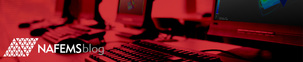### Empty List

Sorry nothing to list